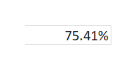# For 2012, the income statement of Precious Metals Processing reported net sales of$17,300, EBIT of$3,450, taxable income of $2,770 and net income of$1,830. Thefirm paid $450 in dividends. The balance sheet reported current assets of$3,650, net fixed assets of $10,850, current liabilities of$1,920, long-term debt of $3,500, common stock of$7,500 and retained earnings of $1,580. Precious Metals Processing is currently operating at full capacity. The profit margin and the dividend payout ratio are held constant. Net working capital and fixed assets vary directly with sales. Sales are projected to increase by 8 percent. What is the external financing needed? Question Asked Feb 12, 2019 127 views For 2012, the income statement of Precious Metals Processing reported net sales of$17,300, EBIT of $3,450, taxable income of$2,770 and net income of $1,830. The firm paid$450 in dividends. The balance sheet reported current assets of $3,650, net fixed assets of$10,850, current liabilities of $1,920, long-term debt of$3,500, common stock of $7,500 and retained earnings of$1,580. Precious Metals Processing is currently operating at full capacity. The profit margin and the dividend payout ratio are held constant. Net working capital and fixed assets vary directly with sales. Sales are projected to increase by 8 percent. What is the external financing needed?

Step 1

Sales, S = $17,300, Current assets, CA =$3,650; Net fixed assets, NFA =  $10,850; Hence, total assets, A = CA + NFA = 3,650 + 10,850 =$ 14,500

Current liabilities, L =  $1,920 Profit Margin = PM = Net income / Sales = 1,830 / 17,300 = 10.58% Dividends = 450; Hence retention ratio, R = 1 - dividend payout ratio = 1 - dividend / net income = 1 - 450 / 1,830 = 75.41%fullscreen Step 2 Sales are expected to increase by 8 percent next year. Hence, change in sales = ΔS = S x growth rate = 17,300 x 8% =$ 1,384

And expected sale in this year = S1 = S + ΔS = 17,300 + 1,384 = \$ 18,684

Step 3

If all assets, short-term liabilities, and costs vary directly with sales, the Additional Fund Needed (AFN) is given by the formula shown on the white board.

...

See Solution

### Want to see this answer and more?

Solutions are written by subject experts who are available 24/7. Questions are typically answered within 1 hour.*

See Solution
*Response times may vary by subject and question.
Tagged in